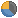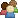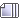Welcome, Guest.

###Stats ezBlockMembers
Total Members: 54
Latest: abrogard
New This Month: 0
New This Week: 0
New Today: 0Stats
Total Posts: 16574
Total Topics: 277
Most Online Today: 97
Most Online Ever: 1155
(April 20, 2021, 12:50:06 pm)Users Online
Members: 1
Guests: 25
Total: 26

###Author Topic: 🚩 Global Climate Chaos ☠️  (Read 92597 times)

0 Members and 0 Guests are viewing this topic.

#### AGelbert##### Re: 🚩 Global Climate Chaos ☠️
« Reply #1380 on: June 27, 2018, 10:15:22 pm »Greater future global warming (still) inferred from Earths recent energy budget

Posted on December 21, 2017 by Patrick T. Brown, PhD (ptbrown31)

We recently published a paper in Nature in which we leveraged observations of the Earths radiative energy budget to statistically constrain 21st-century climate model projections of global warming. We found that observations of the Earths energy budget allow us to infer generally greater central estimates of future global warming and smaller spreads about those central estimates than the raw model simulations indicate. More background on the paper can be obtained from our blog post on the research.

Last week, Nic Lewis published a critique of our work on several blogs titled A closer look shows global warming will not be greater than we thought. We welcome scientifically-grounded critiques of our work since this is the fundamental way in which science advances. In this spirit, we would like to thank Nic Lewis for his appraisal. However, we find Lewis central criticisms to be lacking merit. As we elaborate on below, his arguments do not undermine the findings of the study.

Brief background

Under the emergent constraint paradigm, statistical relationships between model-simulated features of the current climate system (predictor variables), along with observations of those features, are used to constrain a predictand. In our work, the predictand is the magnitude of future global warming simulated by climate models.

We chose predictor variables that were as fundamental and comprehensive as possible while still offering the potential for a straight-forward physical connection to the magnitude of future warming. In particular, we chose the full global spatial distribution of fundamental components of Earths top-of-atmosphere energy budgetits outgoing (that is, reflected) shortwave radiation (OSR), outgoing longwave radiation (OLR) and net downward energy imbalance (N). We investigated three currently observable attributes of these variablesmean climatology, the magnitude of the seasonal cycle, and the magnitude of monthly variability. We chose these attributes because previous studies have indicated that behavior of the Earths radiative energy budget on each of these timescales can be used to infer information on fast feedbacks in the climate system. The combination of these three attributes and the three variables (OSR, OLR and N) result in a total of nine global predictor fields. See FAQ #3 of our previous blog post for more information on our choice of predictor variables.

We used Partial Least Squares Regression (PLSR) to relate our predictor fields to predictands of future global warming. In PLSR we can use each of the nine predictor fields individually, or we can use all nine predictor fields simultaneously (collectively). We quantified our main results with Prediction Ratio and Spread Ratio metrics. The Prediction Ratio is the ratio of our observationally-informed central estimate of warming to the previous raw model average and the Spread Ratio is the ratio of the magnitude of our constrained spread to the magnitude of the raw model spread. Prediction Ratios greater than 1 suggest greater future warming and Spread Ratios below 1 suggest a reduction in spread about the central estimate.

Lewis 🦖 criticism

Lewis post expresses general skepticism of climate models and the emergent constraint paradigm. There is much to say about both of these topics but we wont go into them here. Instead, we will focus on Lewis criticism that applies specifically to our study.

We showed results associated with each of our nine predictor fields individually but we chose to emphasize the results associated with the influence of all of the predictor fields simultaneously. Lewis suggests that rather than focusing on the simultaneous predictor field, we should have focused on the results associated with the single predictor field that showed the most skill: The magnitude of the seasonal cycle in OLR. Lewis goes further to suggest that it would be useful to adjust our spatial domain in an attempt to search for an even stronger statistical relationship. Thus, Lewis is arguing that we actually undersold the strength of the constraints that we reported, not that we oversold their strength.

This is an unusual criticism for this type of analysis. Typically, criticisms in this vein would run in the opposite direction. Specifically, studies are often criticized for highlighting the single statistical relationship that appears to be the strongest while ignoring or downplaying weaker relationships that could have been discussed. Studies are correctly criticized for this tactic because the more relationships that are screened, the more likely it is that a researcher will be able to find a strong statistical association by chance, even if there is no true underlying relationship. Thus, we do not agree that it would have been more appropriate for us to highlight the results associated with the predictor field with the strongest statistical relationship (smallest Spread Ratio), rather than the results associated with the simultaneous predictor field. However, even if we were to follow this suggestion, it would not change our general conclusions regarding the magnitude of future warming.

We can use our full results, summarized in the table below (all utilizing 7 PLSR components), to look at how different choices, regarding the selection of predictor fields, would affect our conclusions.Lewis 🦖 post makes much of the fact that highlighting the results associated with the magnitude of the seasonal cycle in OLR, rather than the simultaneous predictor field, would reduceour central estimate of future warming in RCP8.5 from +14% to +6%. This is true but it is only one, very specific example. Asking more general questions gives a better sense of the big picture:

1) What is the mean Prediction Ratio across the end-of-century RCP predictands, if we use the OLR seasonal cycle predictor field exclusively? It is 1.15, implying a 15% increase in the central estimate of warming.

2) What is the mean Prediction Ratio across the end-of-century RCP predictands, if we always use the individual predictor field that had the lowest Spread Ratio for that particular RCP (boxed values)? It is 1.13, implying a 13% increase in the central estimate of warming.

3) What is the mean Prediction Ratio across the end-of-century RCP predictands, if we just average together the results from all the individual predictor fields? It is 1.16, implying a 16% increase in the central estimate of warming.

4) What is the mean Prediction Ratio across the end-of-century RCP predictands, if we always use the simultaneous predictor field? It is 1.15, implying a 15% increase in the central estimate of warming.

One point that is worth making here is that we do not use cross-validation in the multi-model average case (the denominator of the Spread Ratio). Each models own value is included in the multi-model average which gives the multi-model average an inherent advantage over the cross-validated PLSR estimate. We made this choice to be extra conservative but it means that PLSR is able to provide meaningful Prediction Ratios even when the Spread Ratio is near or slightly above 1. We have shown that when we supply the PLSR procedure with random data, Spread Ratios tend to be in the range of 1.1 to 1.3 (see FAQ #7 of our previous blog post, and Extended Data Fig. 4c of the paper). Nevertheless, it may be useful to ask the following question:

5) What is the mean Prediction Ratio across the end-of-century RCP predictands, if we average together the results from only those individual predictor fields with spread ratios below 1? It is 1.15, implying a 15% increase in the central estimate of warming.

So, all five of these general methods produce about a 15% increase in the central estimate of future warming.

Lewis also suggests that our results may be sensitive to choices of standardization technique. We standardized the predictors at the level of the predictor field because we wanted to retain information on across-model differences in the spatial structure of the magnitude of predictor variables. However, we can rerun the results when everything is standardized at the grid-level and ask the same questions as above.1b) What is the mean Prediction Ratio across the end-of-century RCPs if we use the OLR seasonal cycle predictor field exclusively? It is 1.15, implying a 15% increase in the central estimate of warming.

2b) What is the mean Prediction Ratio across the end-of-century RCPs if we always use the single predictor field that had the lowest Spread Ratio (boxed values)? It is 1.12, implying a 12% increase in the central estimate of warming.

3b) What is the mean Prediction Ratio across the end-of-century RCPs if we just average together the results from all the predictor fields? It is 1.14, implying a 14% increase in the central estimate of warming.

4b) What is the mean Prediction Ratio across the end-of-century RCPs if we always use the simultaneous predictor field? It is 1.14, implying a 14% increase in the central estimate of warming.

5b) What is the mean Prediction Ratio across the end-of-century RCP predictands if we average together the results from only those individual predictor fields with Spread Ratios below 1? It is 1.14, implying a 14% increase in the central estimate of warming.

Conclusion

There are several reasonable ways to summarize our results and they all imply greater future global warming in line with the values we highlighted in the paper. The only way to argue otherwise is to search out specific examples that run counter to the general results.

Appendix: Example using synthetic dataDespite the fact that our results are robust to various methodological choices, it is useful to expand upon why we used the simultaneous predictor instead of the particular predictor that happened to produce the lowest Spread Ratio on any given predictand. The general idea can be illustrated with an example using synthetic data in which the precise nature of the predictor-predictand relationships are defined ahead of time. For this purpose, I have created synthetic data with the same dimensions as the data discussed in our study and in Lewis blog post:

1) A synthetic predictand vector of 36 future warming values corresponding to imaginary output from 36 climate models. In this case, the future warming values are just 36 random numbers pulled from a Gaussian distribution.

2) A synthetic set of nine predictor fields (37 latitudes by 72 longitudes) associated with each of the 36 models. Each models nine synthetic predictor fields start with that models predictand value entered at every grid location. Thus, at this preliminary stage, every location in every predictor field is a perfect predictor of future warming. That is, the across-model correlation between the predictor and the future warming predictand is 1 and the regression slope is also 1.

The next step in creating the synthetic predictor fields is to add noise in order to obscure the predictor-predictand relationship somewhat. The first level of noise that is added is a spatially correlated field of weighing factors for each of the nine predictor maps. These weighing factor maps randomly enhance or damp the local magnitude of the maps values (weighing factors can be positive or negative). After these weighing factors have been applied, every location for every predictor field still has a perfect across-model correlation (or perfect negative correlation) between the predictor and predictand but the regression slopes vary across space according to the magnitude of the weighing factors. The second level of noise that is added are spatially correlated fields of random numbers that are specific for each of the 9X36=324 predictor maps. At this point, everything is standardized to unit variance.

The synthetic datas predictor-predictand relationship can be summarized in the plot below which shows the local across-model correlation coefficient (between predictor and predictand) for each of the nine predictor fields. These plots are similar to the type of thing that you would see using the real model data that we used in our study. Specifically, in both cases, there are swaths of relatively high correlations and anti-correlations with plenty of low-correlation area in between. All these predictor fields were produced the same way and the only differences arise from the two layers of random noise that were added. Thus, we know that any apparent differences between the predictor fields arose by random chance.Next, we can feed this synthetic data into the same PLSR procedure that we used in our study to see what it produces. The Spread Ratios are shown in the bar graphs below. Spread Ratios are shown for each of the nine predictor fields individually as well for the case where all nine predictor fields are used simultaneously. The top plot shows results without the use of cross-validation while the bottom plot shows results with the use of cross-validation.In the case without cross-validation, there is no guard against over-fitting. Thus, PLSR is able to utilize the many degrees of freedom in the predictor fields to create coefficients that fit predictors to the predictand exceptionally well. This is why the Spread Ratios are so small in the top bar plot. The mean Spread Ratio for the nine predictor fields in the top bar plot is 0.042, implying that the PLSR procedure was able to reduce the spread of the predictand by about 96%. Notably, using all the predictor fields simultaneously results in a three-orders-of-magnitude smaller Spread Ratio than using any of the predictor fields individually. This indicates that when there is no guard against over-fitting, much stronger relationships can be achieved by providing the PLSR procedure with more information.

However, PLSR is more than capable of over-fitting predictors to predictands and thus these small Spread Ratios are not to be taken seriously. In our work, we guard against over-fitting by using cross-validation (see FAQ #1 of our blog post). The Spread Ratios for the synthetic data using cross-validation are shown in the lower bar graph in the figure above. It is apparent that cross-validation makes a big difference. With cross-validation, the mean Spread Ratio across the nine individual predictor fields is 0.8, meaning that the average predictor field could help reduce the spread in the predictand by about 20%. Notably, a lower Spread Ratio of 0.54, is achieved when all nine predictor maps are used collectively (a 46% reduction in spread). Since there is much redundancy across the nine predictor fields, the simultaneous predictor field doesnt increase skill very drastically but it is still better than the average of the individual predictor fields (this is a very consistent result when the entire exercise is re-run many times).

Importantly, we can even see that one particular predictor field (predictor field 2) achieved a lower Spread Ratio than the simultaneous predictor field. This brings us to the central question: Is predictor field 2 particularly special or inherently more useful as a predictor than the simultaneous predictor field? We created these nine synthetic predictor fields specifically so that they all contained roughly the same amount of information and any differences that arose, came about simply by random chance. There is an element of luck at play because the number of models (37) is small. Thus, cross-validation can produce appreciable Spread Ratio variability from predictor to predictor simply by chance. Combining the predictors reduces the Spread Ratio, but only marginally due to large redundancies in the predictors.

We apply this same logic to the results from our paper. As we stated above, our results showed that the simultaneous predictor field for the RCP 8.5 scenario shows a Spread Ratio of 0.67. Similar to the synthetic data case, eight of the nine individual predictor fields yielded Spread Ratios above this value but a single predictor field (the OLR seasonal cycle) yielded a smaller Spread Ratio. Lewis post argues that we should focus entirely on the OLR seasonal cycle because of this.However, just as in the synthetic data case, our interpretation is that the OLR seasonal cycle predictor may have just gotten lucky and we should not take its superior skill too seriously.https://patricktbrown.org/2017/12/21/greater-future-global-warming-still-inferred-from-earths-recent-energy-budget/

Smileys added by Agelbert. Don't blame Dr. Brown!Light is sown for the righteous, and gladness for the upright in heart. Ps. 97:11

###Recent Topics

 COVID-19 🏴☠️ Pandemic by AGelbert October 20, 2021, 01:28:39 pm Homebody Handy Hints by AGelbert October 19, 2021, 07:06:26 pm The Big Picture of Renewable Energy Growth by AGelbert October 19, 2021, 06:10:06 pm Electric Vehicles by AGelbert October 19, 2021, 05:23:18 pm Christian Teachings by AGelbert October 18, 2021, 09:19:49 pm Batteries by AGelbert October 16, 2021, 09:32:13 pm Majestic and sometimes Spectacular Scenery by AGelbert October 16, 2021, 08:36:09 pm Defending Wildlife by AGelbert October 16, 2021, 07:54:14 pm Pollution by AGelbert October 14, 2021, 04:47:46 pm TRULY AFFORDABLE self-navigating cane for visually impaired by AGelbert October 14, 2021, 04:27:24 pm